# 并发算法理论

[toc]

## 内存模型

### Sequential Consistency model

n个线程一个共享的内存memory，每一个读都是最近的写入的值；（交错，通过枚举行为得到所有可能的行为）

SC是比较强的内存模型；比如如下例子：

Init x=y=0
x=1 \ \ y=1
r1=y \ \ r2=x

## 弱内存模型

### 设计标准

1. DRF(data race freedom) guarantee 数据竞争保证：满足DRF的程序在弱内存模型下的行为和SC模型的行为一样；（其中怎么看待一个程序是不是DRF的指的是这个程序在SC下面是不是DRF的）

DRF定义：

当出现两个并发冲突操作的时候会出现数据竞争：

1. 冲突：两个操作都访问一块内存区域并且至少一个是写操作
2. 并发：
1. 在不同模型不一样
2. 在java里面，就是两个操作没有happen-before顺序；
1. 如果是SC model；那么一个线程内部前后操作c1,c2；或者两个线程的通过锁保护的部分有happen-before关系；(也就是 $program ~order~\cup~synchronize-with$)
2. 可以被实现(不能特别强)

1. 优化：支持通常的优化（编译器的优化）
2. 硬件：允许正常的map到现代体系结构的compilation scheme
3. 保证内存安全和安全保证（不能特别弱）,不能出现一些凭空出现的行为

### java memory model的核心——happen-before memory model(hmm)

1. 相对于SC的枚举定义行为的方式，HMM使用声明式(declarative semantics)定义程序的行为（给一个行为判定是不是满足weak memory model约束）

#### Happen before order

1. 程序执行：事件集合，他们之间存在顺序(po(program order)+sw(synchronize-with)):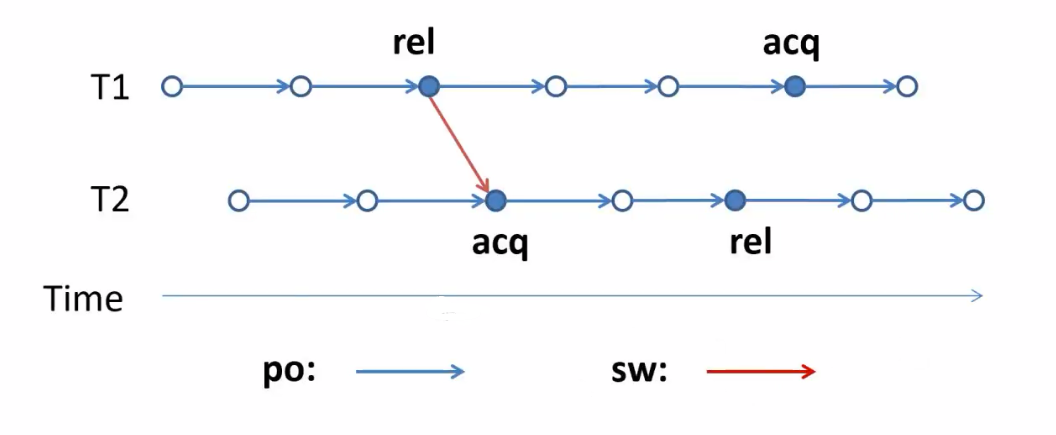2. happen before order:$po\cup sw$的传递闭包: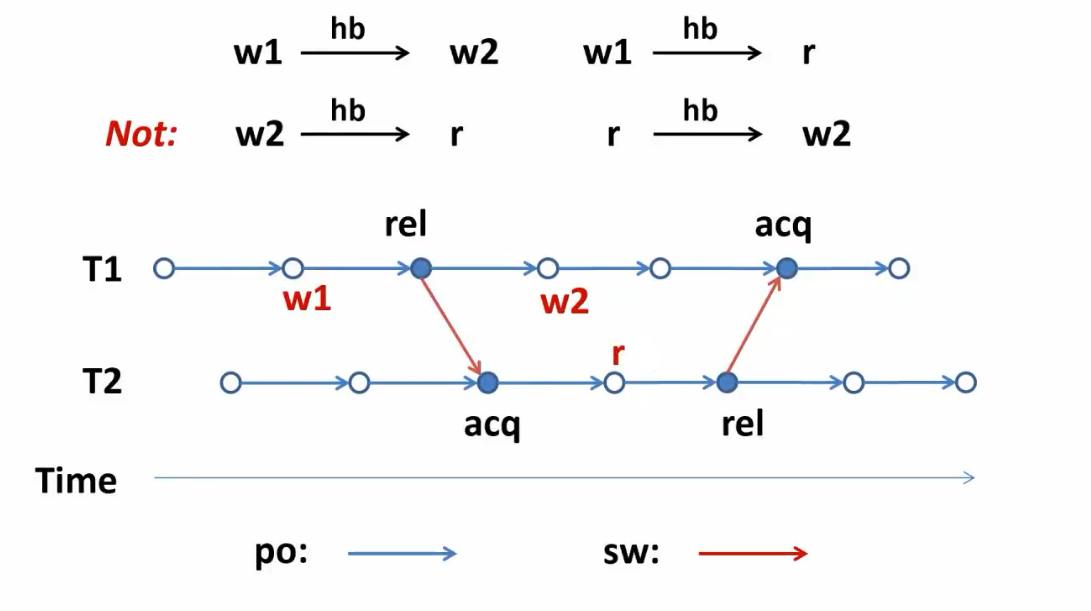1. 在它之前happen-before最近的写
2. 与他毫无happen-before的写

上图中r可以看到w1也可以看到w2

4. 判定程序行为的方式：

1. 画执行图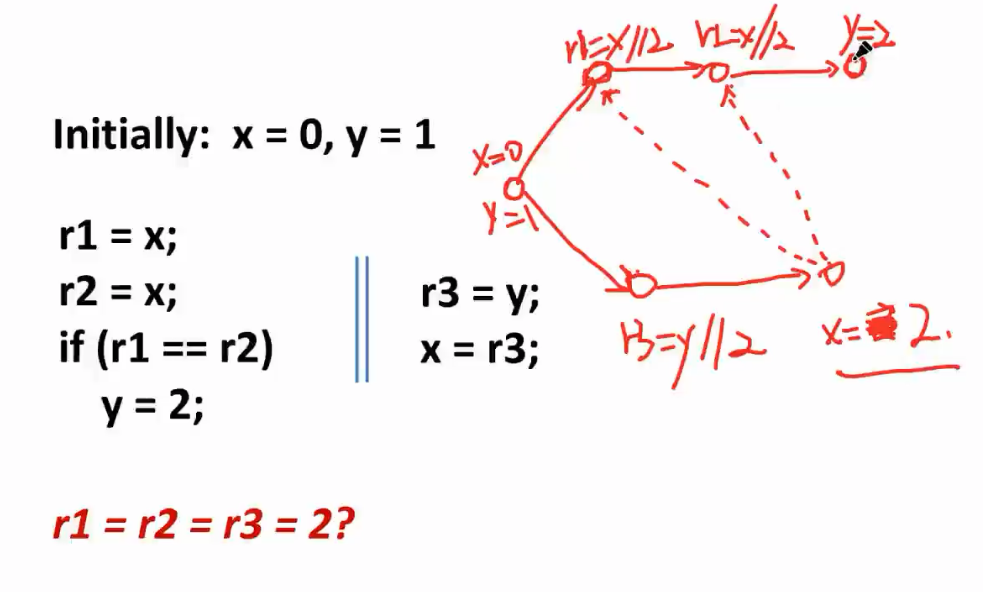2. 给一个行为，判断合不合理（先猜然后解读，形成环）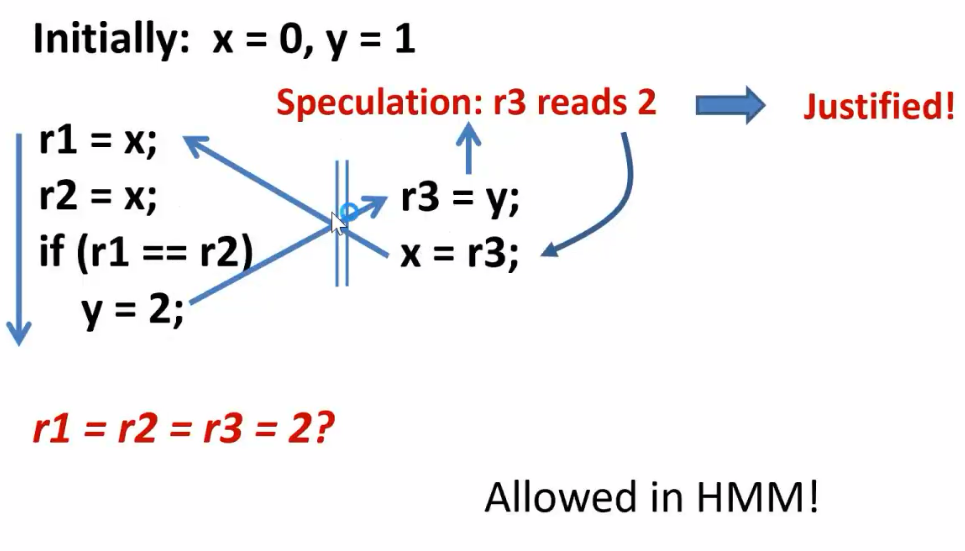## 并发的基本操作语义

### basic domain

1. $r\in Reg$ 表示寄存器，本地变量
2. $x\in Loc$ 共享的，地址
3. $v\in Val$ 值，包含0
4. $i\in Tid={1,..,N}$ 线程标识

### Expressions and commands

1. $e::=r|v|e+e|e-e|e*e|e/e$
2. $c::=skip|if~e~then~c~else~c|while~e~do~c|c;c|r:=e|r:=x|x:=e|r:=FAA(x,e)|r:=CAS(x,e,e)|fence$
4. CAS(x,e,e):Compare-and-Swap:比较memory里面的东西是否和前面一个e一样，如果一样就设置为新的值(后面的e)，如果不一样就什么都不做；这也是原子操作；返回值表示是否成功(成功r为1否则为0)
5. fence: SC下啥都不做；weak memory model里面x=1;fence;y=x;fence会保证x一定已经写入了memory；

### Program

$P:Tid\rightarrow Cmd,written ~as~P=c_1||…||c_N$

### 描述程序含义

$P,S,M\Rightarrow P’,S’,M‘$

S表示线程的寄存器集合，M表示共享内存，P表示程序；

#### 线程子系统简述

1. 线程代码c， 自己的寄存器是s:$c,s\stackrel{l}{\longrightarrow}c’,s’$
2. 顺序程序
3. 提升到程序步骤:$P,S\stackrel{i:l}{\longrightarrow}P’,S’$

#### 存储子系统简述

1. 描述内存访问以及fence
2. $M\stackrel{i:l}{\longrightarrow}M’$

### 线程子系统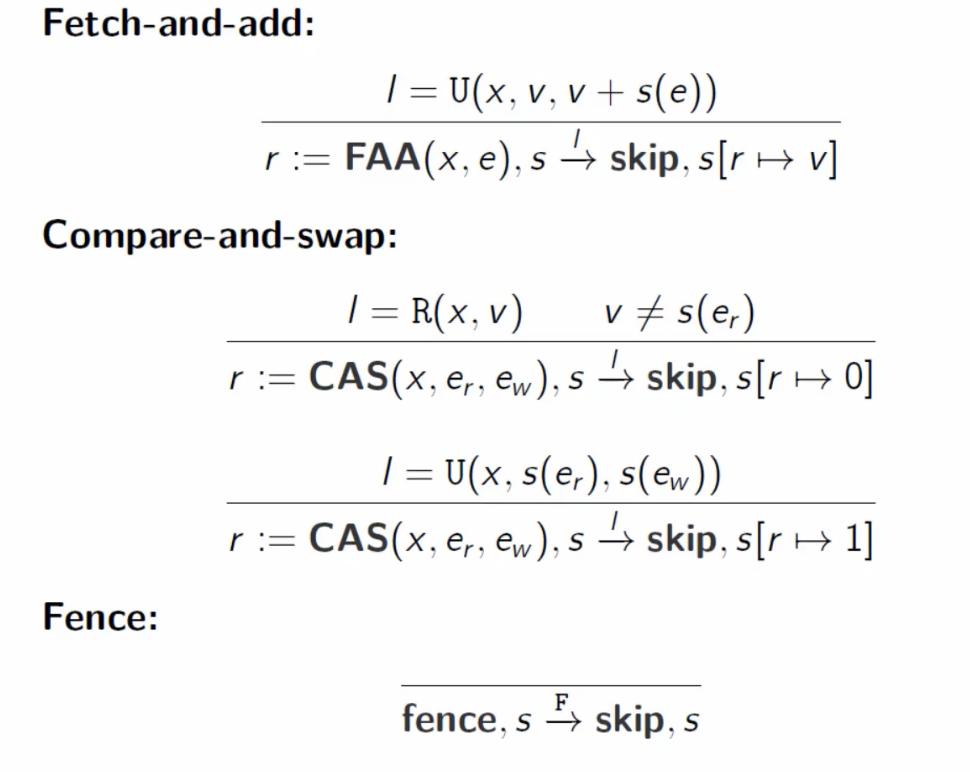U(x,v,v+s(e))表示update，既可以读memory也可以写memory；

#### 合成到并发程序级别

Transition表示只有线程i相关的部分(程序和register)发生了变化，表示：每次都是任取一个线程i走一步，然后代码就是线程i执行之后的结果，其他线程的部分不变

### 存储子系统

#### Sequential Consistency model 存储子系统

1. SC model：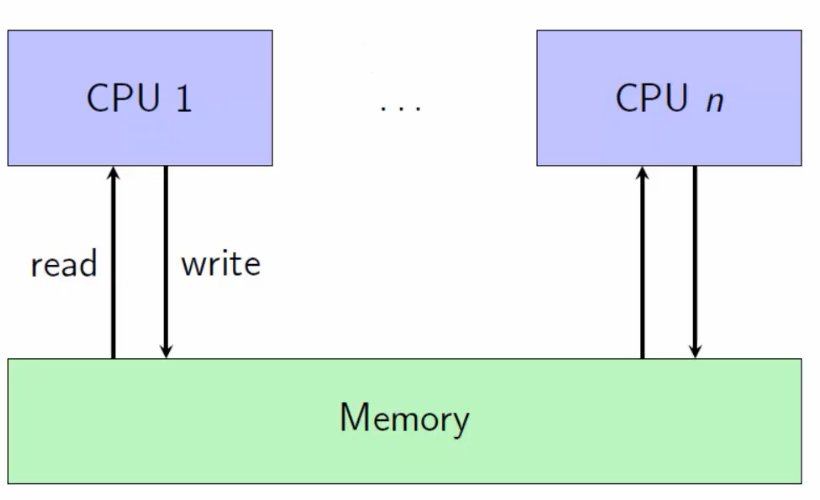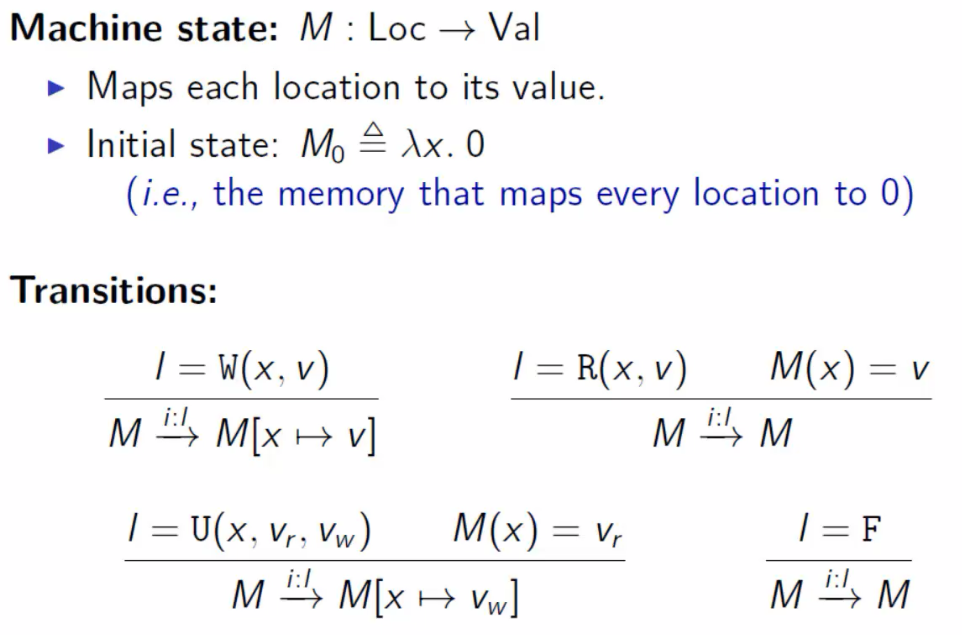### TSO 存储子系统

1. TSO存储模型，用于x86：

每一个线程都有一个buffer，写的时候不会直接写而是写到buffer里面；然后不定时的把清空buffer，把里面的值写入到memory里面；读的时候先看buffer，有的话直接读取buffer否则读memory；

存在情况，线程A写到了自己的buffer里面，线程B读的是memory里面的值；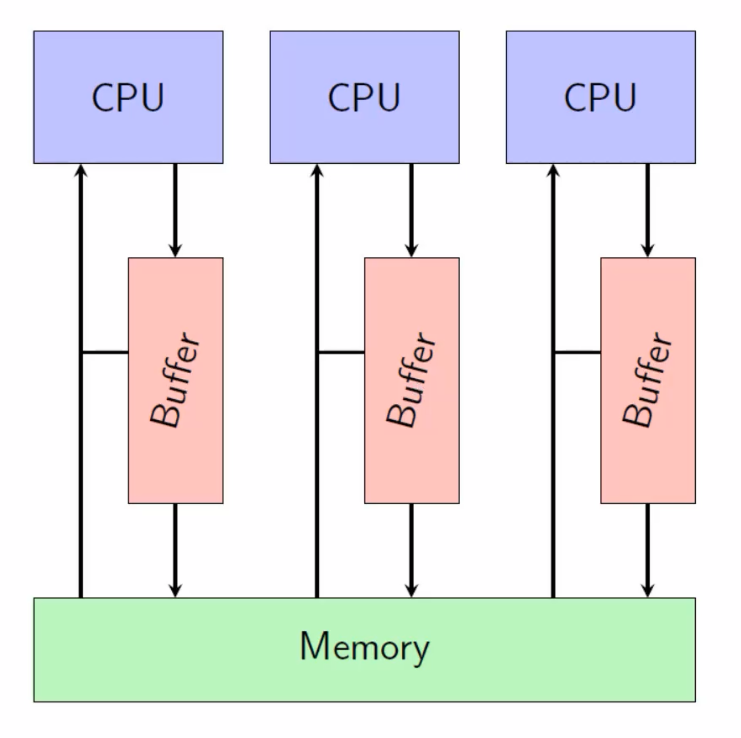相对于上面只有一个接口l，现在下面这部分需要考虑buffer和memory这块接口以及把他们合起来的方法；

2. TSO存储子系统的状态：

1. A memory M:$Loc\rightarrow Val$ 通过Loc可以获得值
2. A function B: $Tid\rightarrow (Loc,Val)^*$ 每一个线程都有自己的buffer，buffer里面是一串写操作(通过(Loc，Val)对表示)；

初始状态：$<M_0,B_0>$

1. $M_0=\lambda x.0$ 所有位置都是0
2. $B_0=\lambda i.\epsilon$ 所有buffer都是空的

3. TSO存储子系统的状态转化：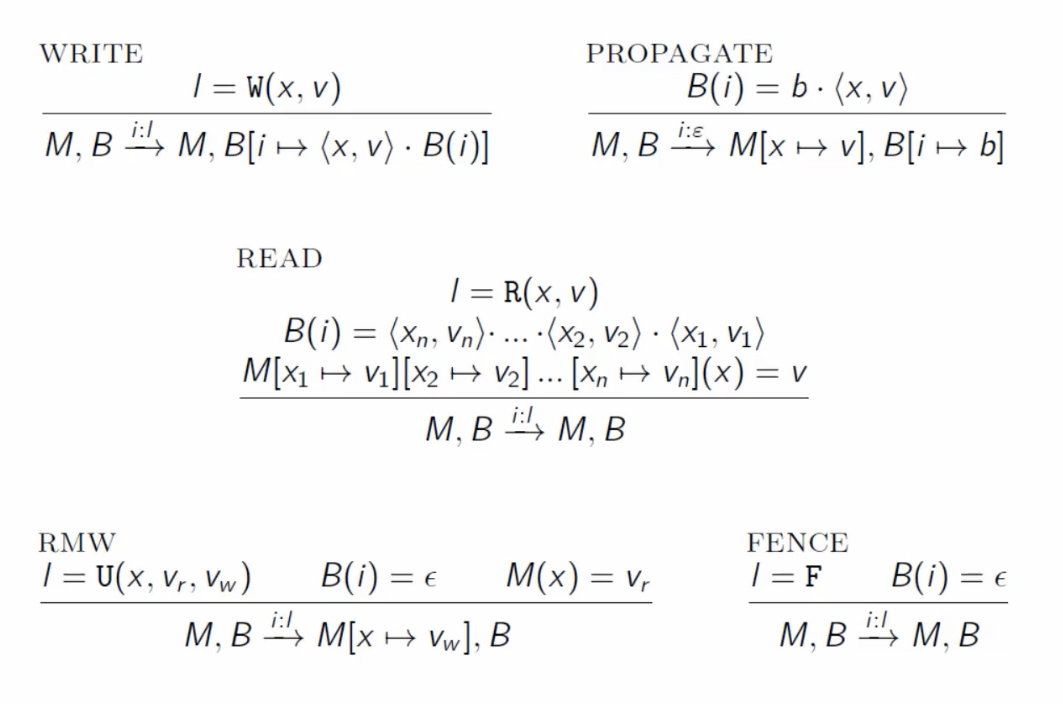WRITE表示在原来的基础上增加一个$<x,v>$操作；

PROPAGATE表示把buffer里面的内容写入memory；buffer里面越往右越旧，所以先写右边的内容；

FENCE 这个操作要求当前线程的buffer是空的；

4. TSO模型的例子:

| 例子 | store | buffering |
| —- | —– | ——— |
| x=1 | || | y=1 |
| r1=y | || | r2=x |

r1=r2=1?

TSO模型可以（两边都读的旧值），SC模型不行

## 并发的声明式语义(Declarative semantics for concurrency)

1. 总体思想：不去想程序怎么执行（操作语义这么整），而是产生一系列程序有可能执行的图，然后通过规则判定这些图上哪一些能够被model解释

2. 具体而言：

1. Define the notion of a program execution (generalization of an execution trace)
2. Map a program to a set of executions (与程序有关)
3. Define a consistency predicate on executions (定义公理，比如happen-before等约束)
4. Semantics = set of consistent executions of a program (上述满足约束的所有程序)
3. 例子：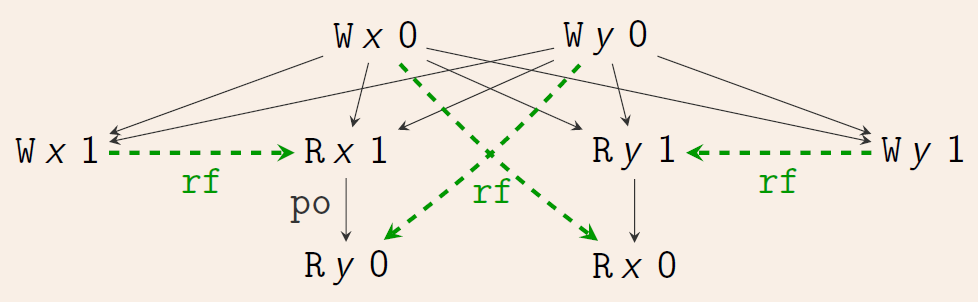2. 有两种关系：程序执行顺序(Program order,po;或者“sequenced-before”, sb)，黑色实线; Reads-from 顺序, rf，绿色虚线

### 执行图的定义

1. Label：有以下格式：

$R~x~v_rW~x~v_w U(x~v_r~ v_w) ~~F$

其中$x \in Loc$ and $v_r , v_w \in Val$.

2. Event：是一个元组$<id, i, l>$:

1. $id\in N$表示事件标识符
2. $i \in Tid \cup {0}$ 是线程标识符
3. l是一个label
3. 执行图(execution graph)：是一个元组$<E, po, rf>$：

1. E是events的有限集合

2. po (“program order”) 是 E 上的偏序关系

3. rf (“reads-from”) 是 E 上的二元关系：

For every $<w, r>\in rf$:

1. $typ(w) \in {W, U}$ w操作要么是写要么是更新(U)
2. $typ(r) \in {R, U}$ r操作要么是读要么是更新
3. $loc(w) = loc(r)$ 读写的位置一样
4. $val_w(w) = val_r(r)$ 读写的值一样

$rf^{ −1}$ is a function,that is: if $<w_1, r>$, $<w_2, r>$,then $w_1 = w_2$ ；表示读只能读一个写

4. 其他一些标记：### 程序映射到执行图

1. 例子，与程序有关的图：2. 线程子系统将一个顺序(sequential)执行图与每个命令关联起来。通过连接组成线程的顺序执行图可以得到程序的执行；

如果满足以下性质，一个图是sequential的（也就是与一个线程有关的执行图的性质）：

1. $tid(a) = 0$ for every $a \in G.E$ （与下面第四点Thread restriction有关，表示只有一个线程）
2. $G.po$ is a total order on $G.E$ （一个线程中的事件一定是有序的）
3. $G.rf = \empty$
3. 命令的执行图的定义：与内存有交互的命令会产生执行图的变化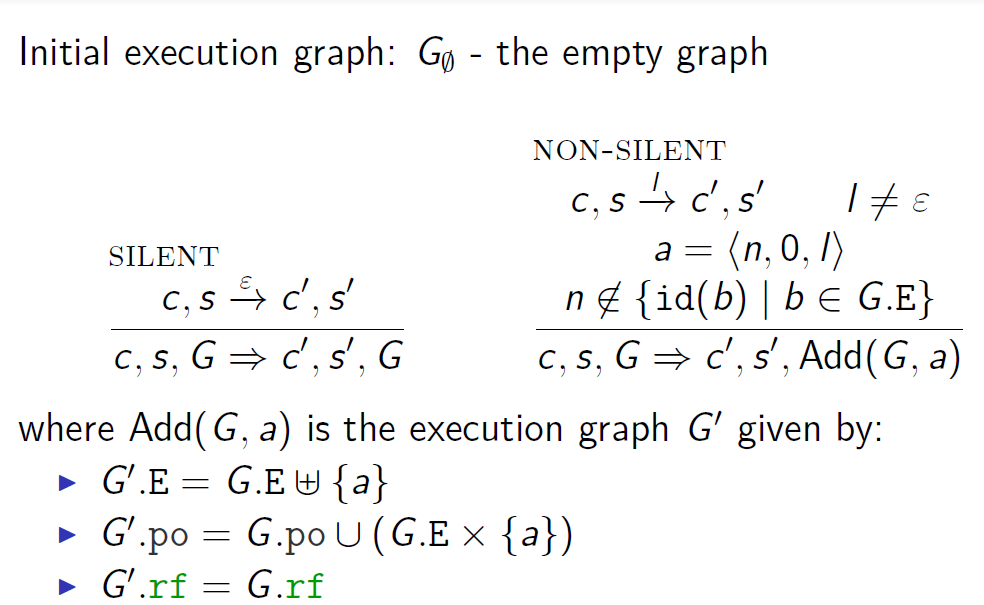给定线程号$i\in Tid$，以及一个执行图G，$G^i$表示把G的事件限制成只有与线程i相关的事件:${a\in G.E|tid(a)=i}$，并且把他们的线程标识都改成0，丢弃所有rf边；

5. Execution graph of a program:

$G$ is an execution graph of a program $P$ (with an outcome $O)$ if $G_i$ is an execution of $P(i)$ (with final store O(i)) for every
$i \in Tid$.

### 一致性谓词(consistency predicate)

1. 假设X是执行图上的一些一致性谓词，那么程序P在X下允许出现的结果O，定义为如果存在一个执行图G满足：

1. G is an execution graph of P with outcome O. G是P的执行图能产生O
2. G is X-consistent. G满足X-一致性

或者，“catch-fire” semantics定义：(对应于C/C++的undefined behavior，此时任何的O都可以产生)

存在一个执行图G满足：

1. G is an execution graph of P. G是P的执行图
2. G is X-consistent. G满足X-一致性
2. Completeness定义

#### Sequential consistency

1. SC-consistent 定义：假设sc是G,E上面的全序；如果下列情况满足，则称G是关于sc SC -consistent的：

1. If $<a, b> \in G.po$ then $<a, b>\in sc$. (program order 里的都满足sc)
2. If $<a, b> \in G.rf$ then $<a, b> \in sc$ and there does not exist $c \in G.W_{loc}(b)$ such that $<a, c> \in sc$ and $<c, b> \in sc$. (不同线程之间读应该读上一次写，中间有write是不可能的)
2. 一个执行图如果满足下列条件那么是SC-consistent的：

1. G is complete.
2. G is SC-consistent wrt some total order sc on G.E. (所有事件之间要有一个sc order)
3. 例子：forbidden部分因为sc下一定会形成一个环，此时这个不是order了，因此不允许；

allowed部分可以先R y 0然后W y 1，此时就可以构成全序了；

#### Sequential consistency 另外的定义

1. Modification order定义:

$mo$ is called a modification order for an execution graph G if $mo = \cup _{x\in Loc} mo_x$ where each $mo_x$ is a total order on $G.Wx$ .

mo只在这张图的write之间建立，同一个loc上的write上面有一条边，称为$mo_x$，所有$mo_x$的并形成了mo；

2. SC的另一种定义：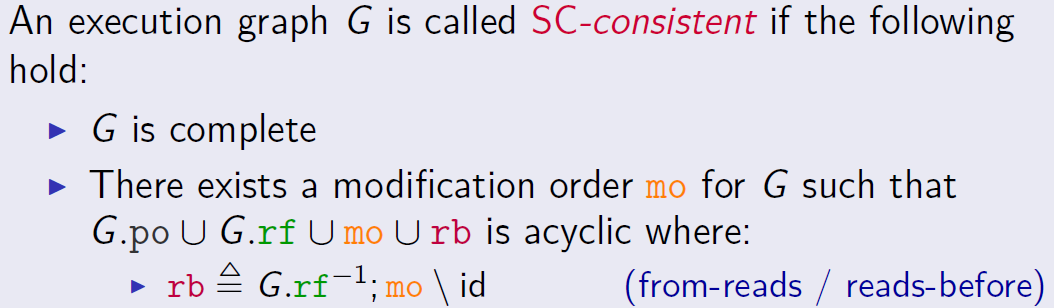所有的po,rf,mo,rb边合起来是无环的；

rb的定义就是rf取逆然后加上mo，比如$Wy0 \rightarrow Wy1 (mo),Wy0\rightarrow Ry0(rf)$，因此$Ry0\rightarrow Wy0(rf^{-1})$，有$Ry0\rightarrow Wy1(rb)$

rb的定义去掉id是考虑到U的情况（因为U和W可以有mo关系也可以有rf关系，有可能形成自环）

#### Relax Sequential consistency

1. 大多数硬件保证SC-per-location，又称作coherence；如果一个执行图是coherent的那么有如下性质：

1. G is complete

2. 对于所有的位置x，在所有对x的访问上存在一个全序关系$sc_x$:

1. if $<a,b>\in [RW_x];G.po;[RW_x]$,then $<a,b>\in sc_x$

$Rw_X$表示对x的读写操作;

$<a,b>\in A;B$:这条的意思是a,b是和x的读写有关的，如果它们之间是program order的关系，那么它们要属于$sc_x$

2. If $<a, b> \in [W_x];G.rf; [Rx]$ , then $<a, b> \in sc_x$ and there does not exist $c \in G.W_x$ such that $<a, c> \in sc_x$ and
$<c, b> \in sc_x$ .

与前面的定义相似，主要区别是对于x的位置限定

2. 另外一个版本的定义：主要区别是在同一个location上面不能成环；

反例：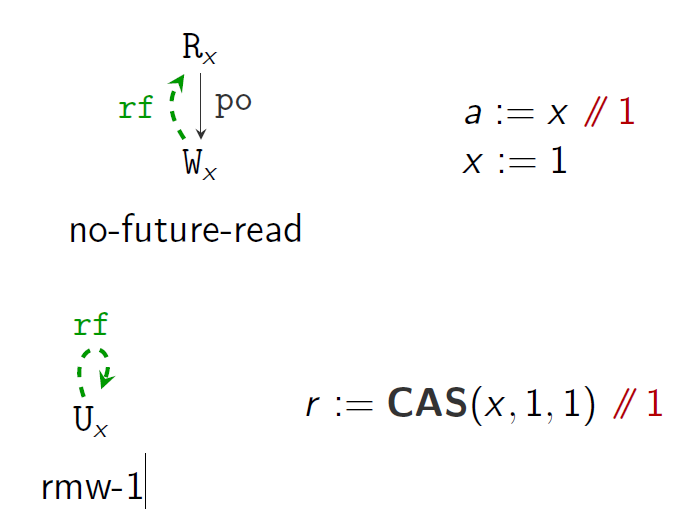2. coherence-ww,coherence-rw,coherence-wr,coherence-rr:

下面程序对应于最后一个执行图，是不行的（有环）3. rmw-2,atomicity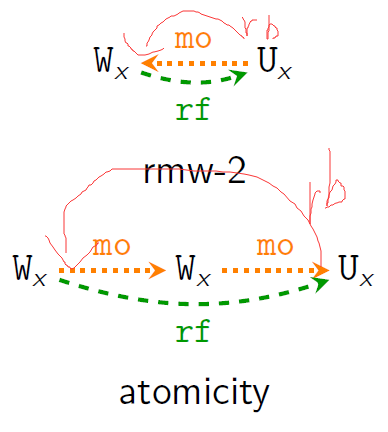3. 另外一个版本的定义（对应于上面这些图）：

假设mo是执行图G的modification order，那么G关于mo是coherent的当且仅当以下性质成立：

1. rf; po is irreflexive. (no-future-read)
2. mo; po is irreflexive. (coherence-ww)
3. mo; rf; po is irreflexive. (coherence-rw)
4. rf−1; mo; po is irreflexive. (coherence-wr)
5. rf−1; mo; rf; po is irreflexive. (coherence-rr)
6. rf is irreflexive. (rmw-1)
7. mo; rf is irreflexive. (rmw-2)
8. rf−1; mo; mo is irreflexive. (rmw-atomicity)

4. coherence相对还是太弱了，没有办法让锁真正应用（因为lock之后对于其他地方的修改其实该一致性不做限制）

#### Release/acquire (RA) memory model

1. 注：$(a,b)\in (po\cup rf)^+$ 后者表示闭包，也就是a,b之间po,rf边出现一次或者多次；实际上比po要延续更长的距离，让model更强

2. RA实际上就是把coherence的po换成了$(a,b)\in (po\cup rf)^+$: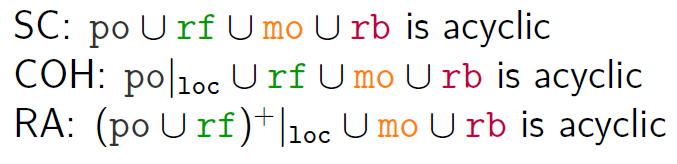3. 具体定义：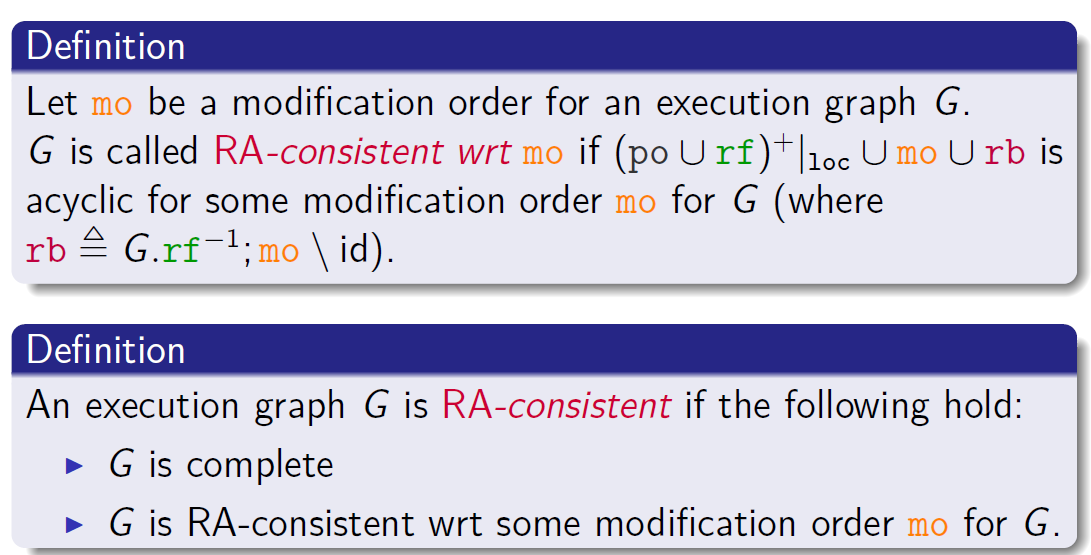4. 另一种定义：

1. $(po \cup rf)^+$ is irreflexive. (no-future-read) 也就是po,rf无环；不会是某一个事件a开始经过若干个po,rf然后回到a；
2. $mo; (po \cup rf)^+$ is irreflexive. (coherence-ww) 不会是某一个事件a开始首先经过mo然后经过若干个po,rf然后回到a；
3. $rf^{−1}; mo; (po \cup rf)^+$ is irreflexive. (coherence-wr)
4. $rf^{−1}; mo; mo$ is irreflexive. (rmw-atomicity)

#### Access model

1. 每一个内存访问都有一个模式：

2. Writes: rlx or rel
3. RMWs: rlx, acq, rel or acq-rel

PS: rl 是 relax的缩写，指的是满足coherence model一致性；rel,release缩写，acq,acquire缩写，满足RA memory model一致性

2. 强度顺序,$\sqsubset$定义：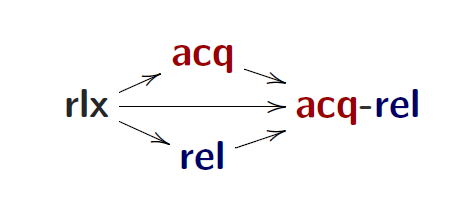中间的表示读是rlx然后写不一样

##### Happen before
1. 同步(Synchronization)：

表示的是W要么是release要么比release更强；R同理

2. Happen-before: $G.hb = (G.po \cup G.sw)^+$

#### C/C++11 memory model 简介

1. 定义：实际上是把coherence model的po用hb进行了”增强”：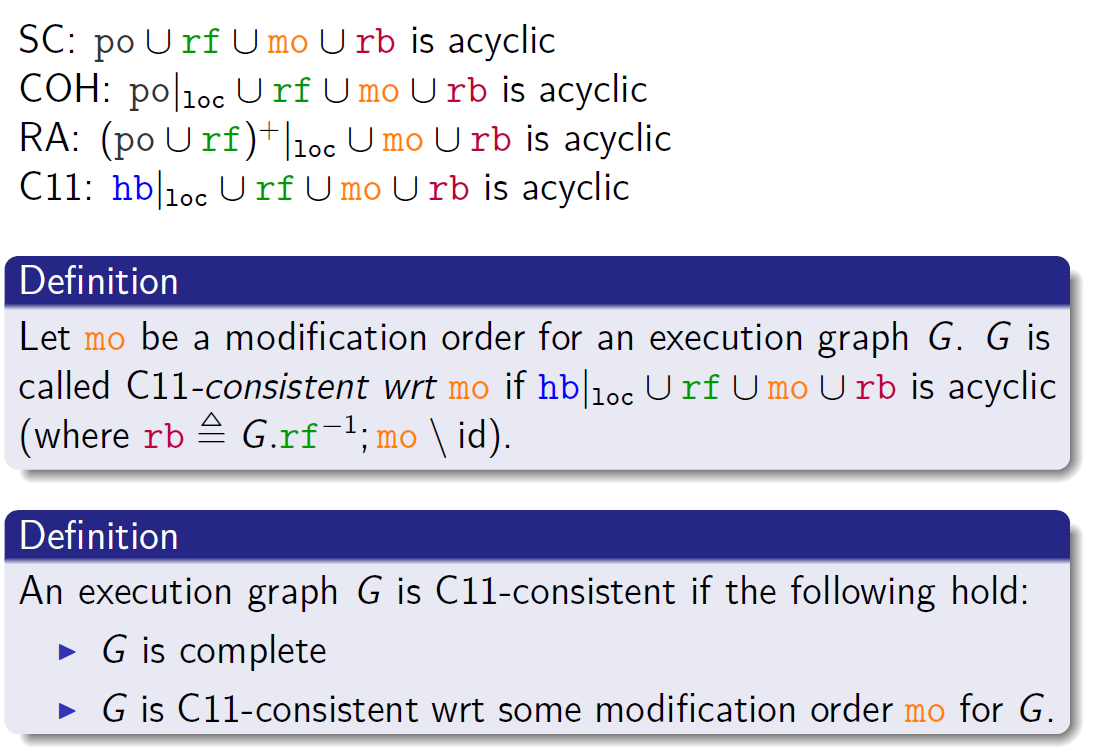2. C/C++11 memory model 下面的强度关系：(比access model 更多一点类型)

$non-atomic\sqsubset relaxed\sqsubset release/acquire\sqsubset sc$

1. non-atomic用于没有数据竞争的代码（默认）
2. fence也有以上四种类型